Ex 4.3

Chapter 4 Class 8 Practical Geometry
Serial order wise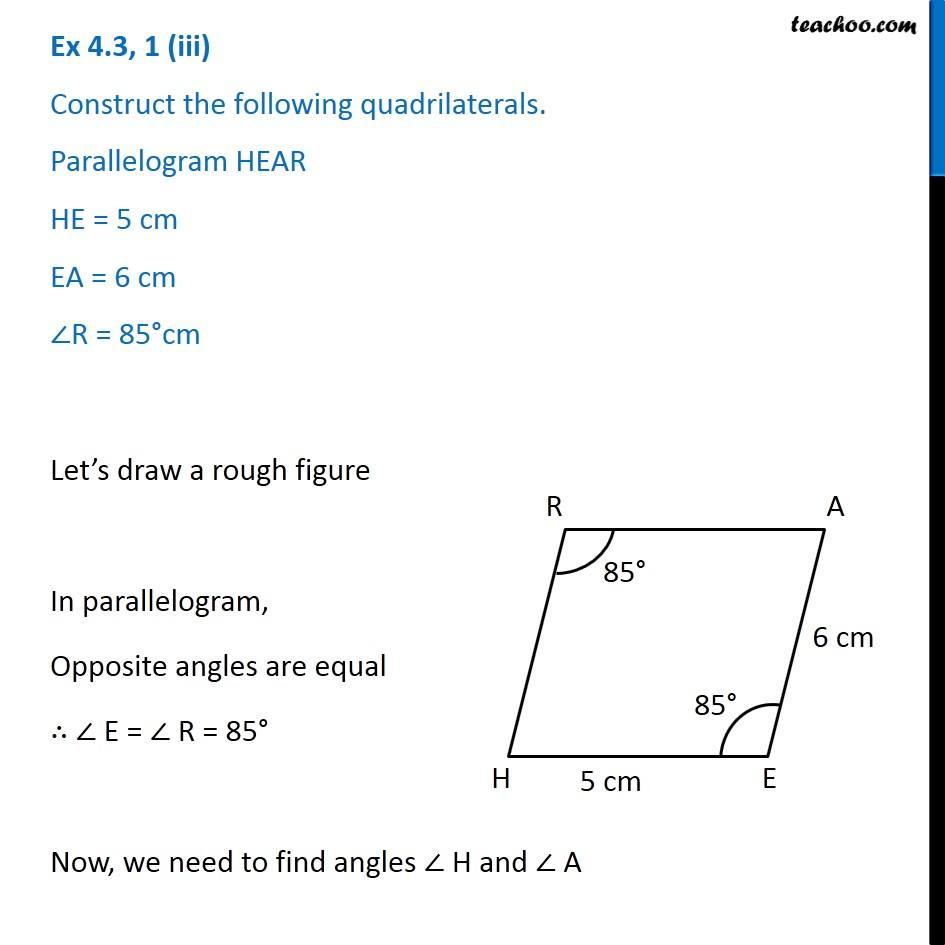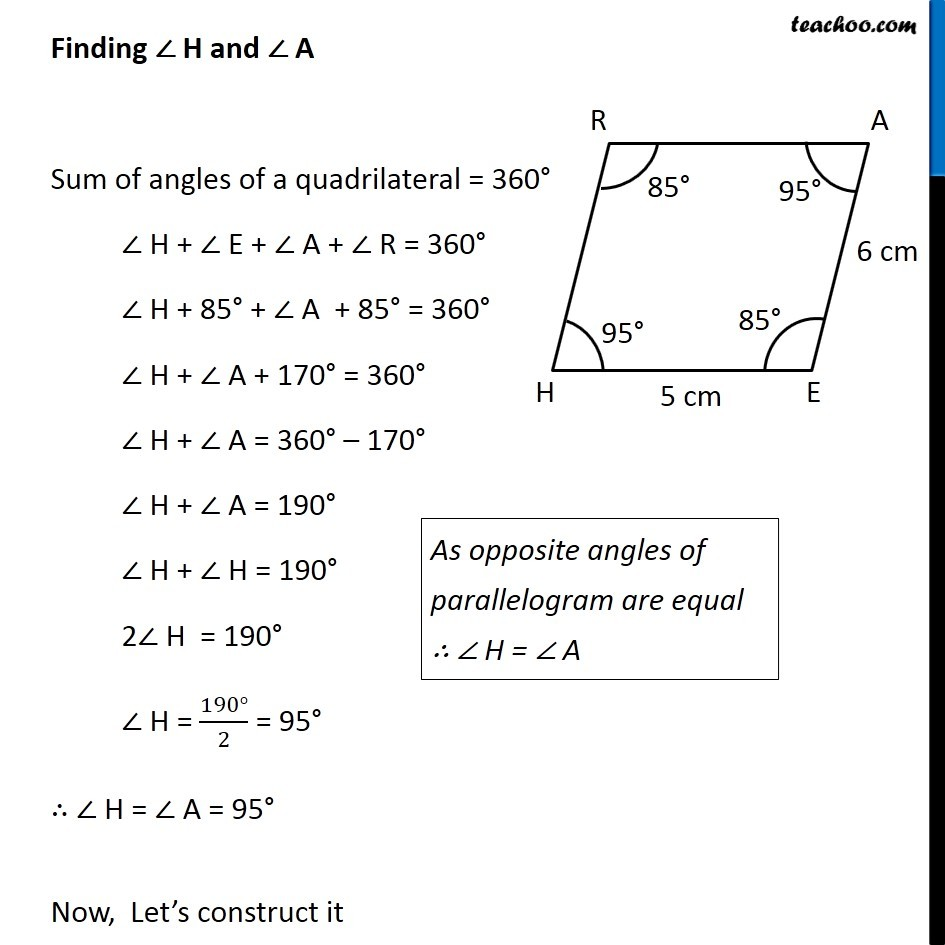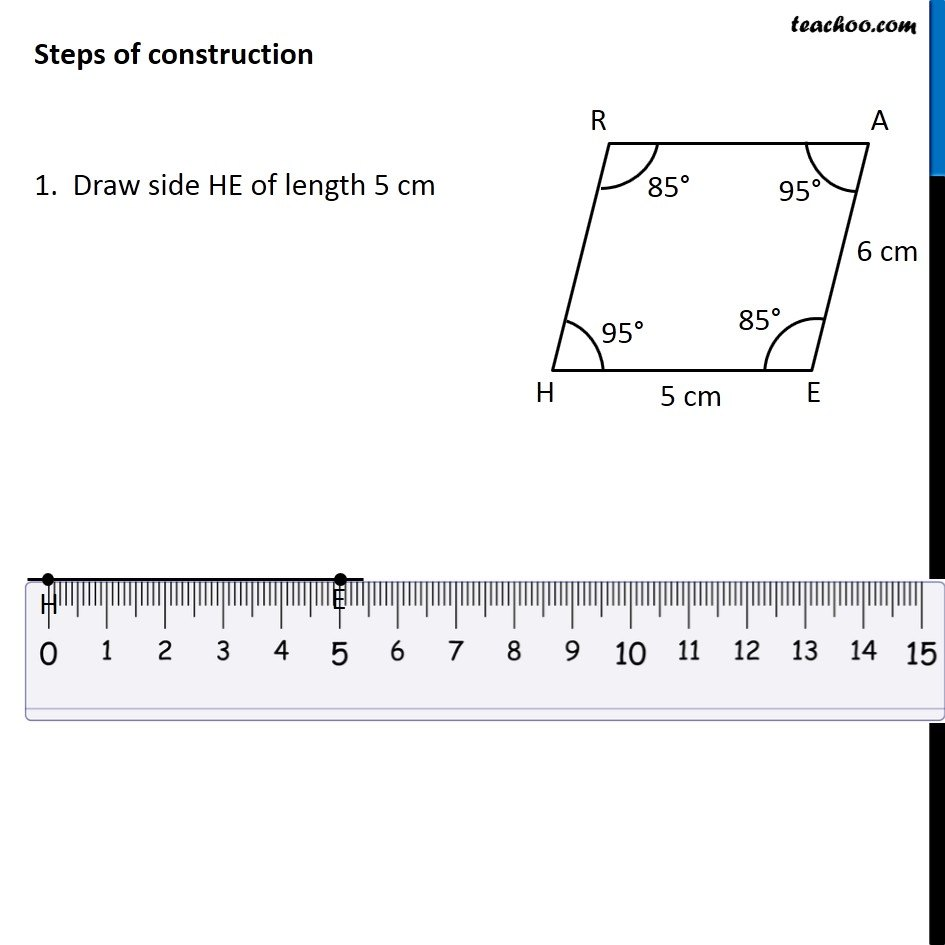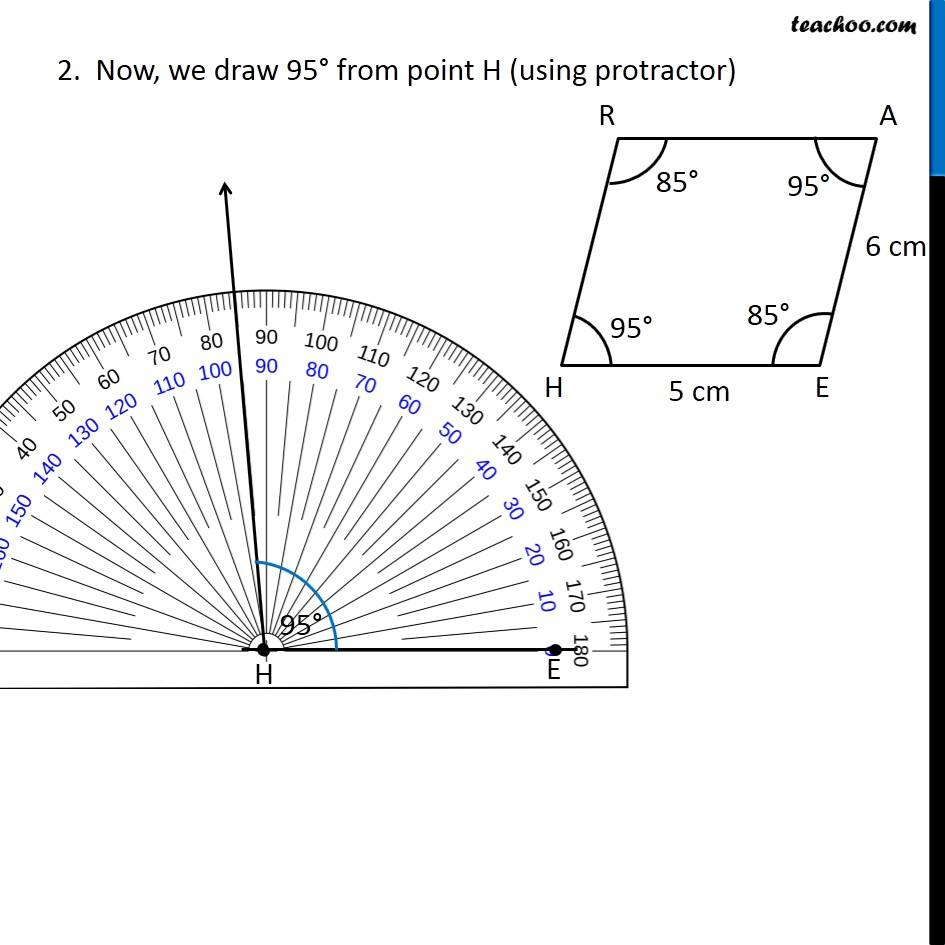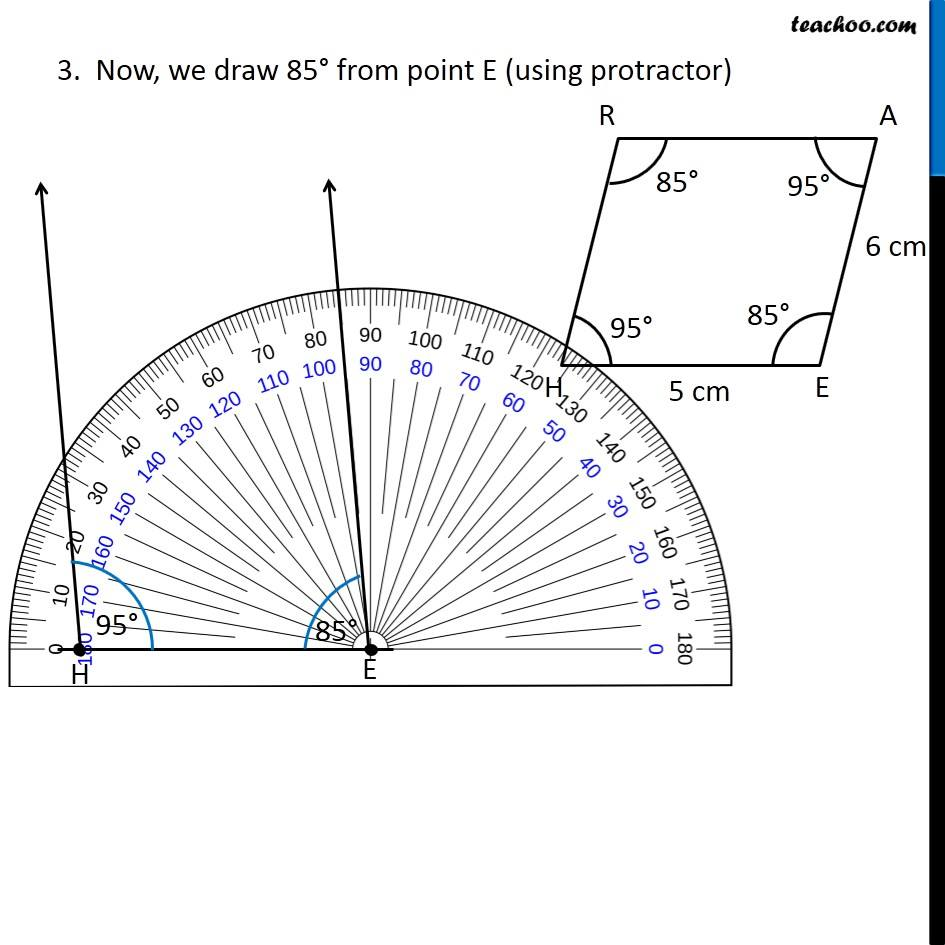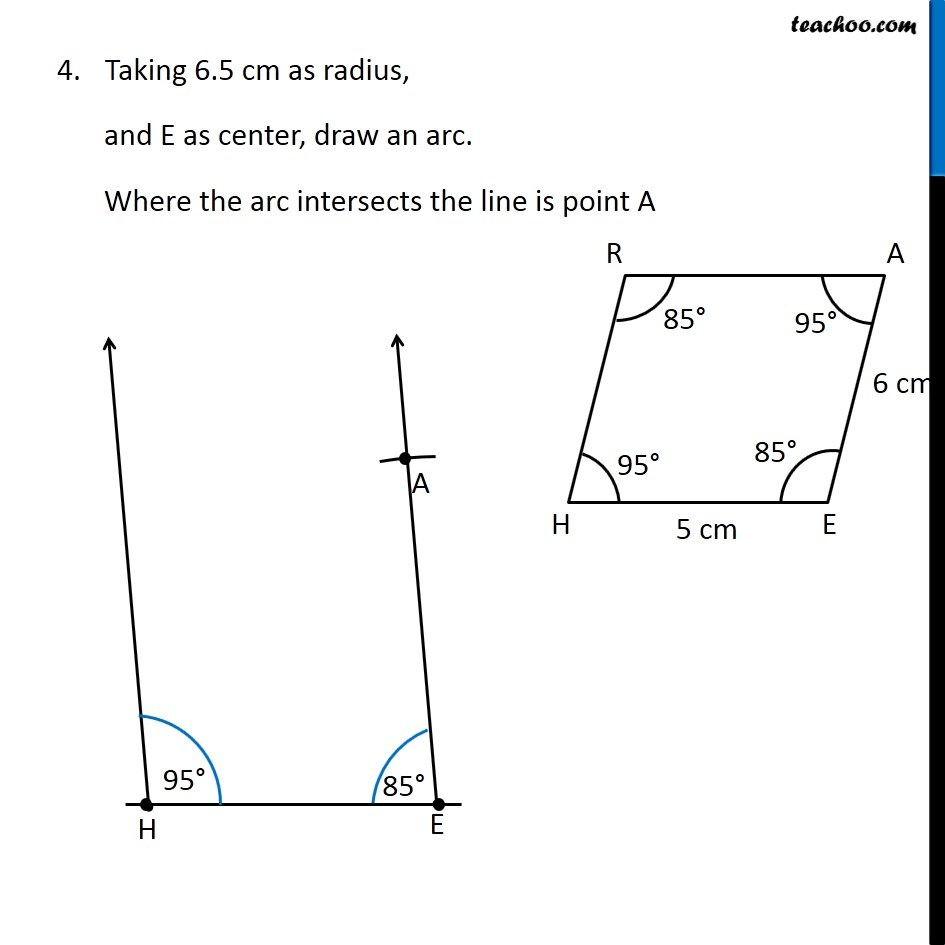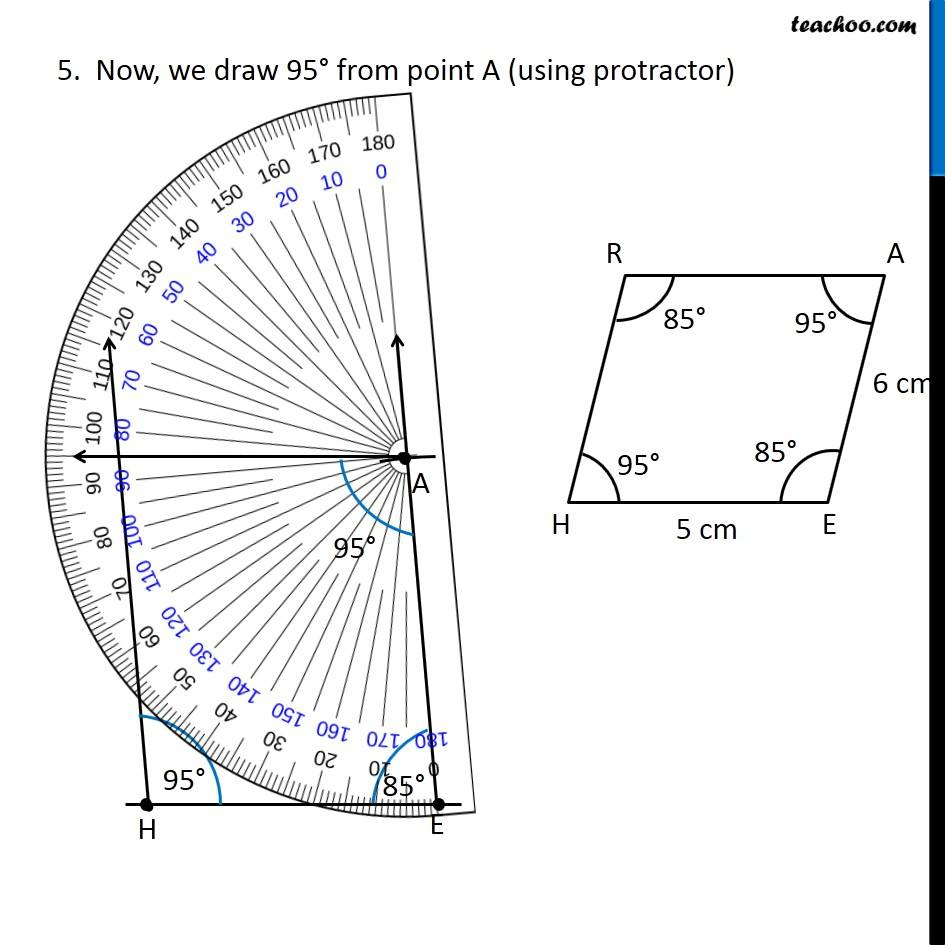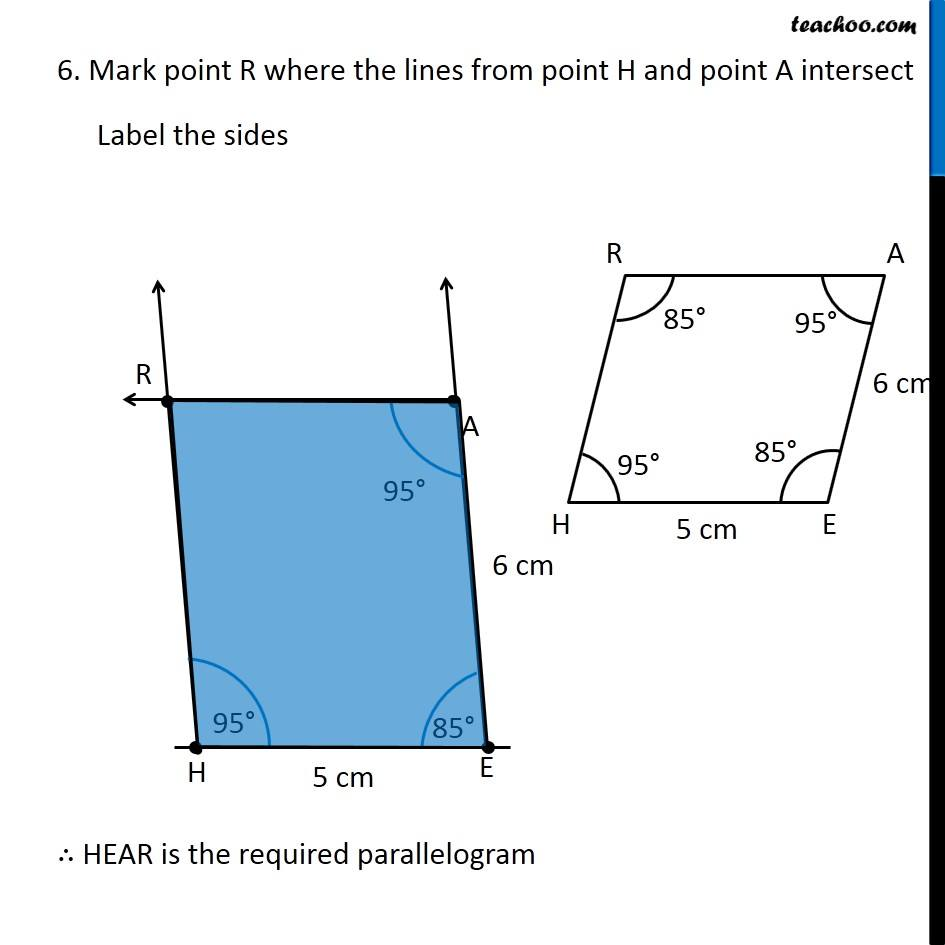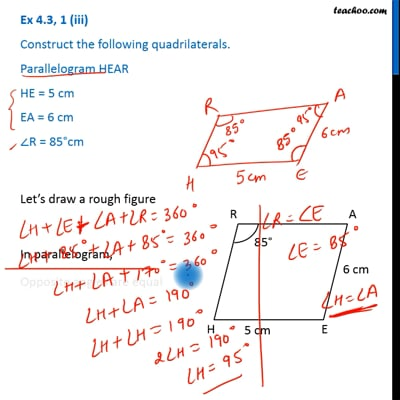This video is only available for Teachoo black users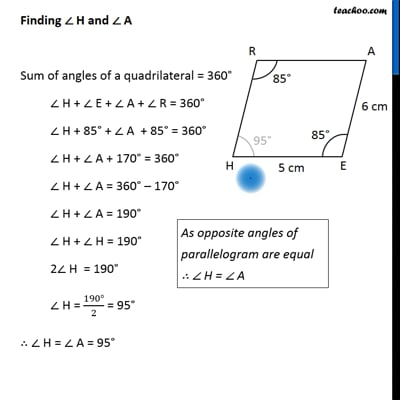This video is only available for Teachoo black users

Get live Maths 1-on-1 Classs - Class 6 to 12

### Transcript

Ex 4.3, 1 (iii) Construct the following quadrilaterals. Parallelogram HEAR HE = 5 cm EA = 6 cm ∠R = 85°cm Let’s draw a rough figure In parallelogram, Opposite angles are equal ∴ ∠ E = ∠ R = 85° Now, we need to find angles ∠ H and ∠ A Finding ∠ H and ∠ A Sum of angles of a quadrilateral = 360° ∠ H + ∠ E + ∠ A + ∠ R = 360° ∠ H + 85° + ∠ A + 85° = 360° ∠ H + ∠ A + 170° = 360° ∠ H + ∠ A = 360° – 170° ∠ H + ∠ A = 190° ∠ H + ∠ H = 190° 2∠ H = 190° ∠ H = (190°)/2 = 95° ∴ ∠ H = ∠ A = 95° Now, Let’s construct it As opposite angles of parallelogram are equal ∴ ∠ H = ∠ A Steps of construction 1. Draw side HE of length 5 cm 2. Now, we draw 95° from point H (using protractor) 3. Now, we draw 85° from point E (using protractor) Taking 6.5 cm as radius, and E as center, draw an arc. Where the arc intersects the line is point A 5. Now, we draw 95° from point A (using protractor) 6. Mark point R where the lines from point H and point A intersect Label the sides ∴ HEAR is the required parallelogram